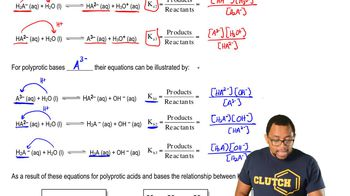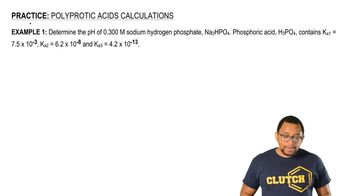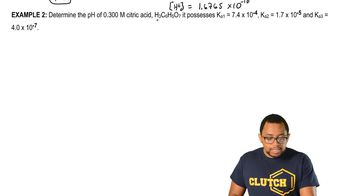Start typing, then use the up and down arrows to select an option from the list.# Polyprotic Acids

Jules Bruno
202views
1
our understanding of die product acids and bases can be used to understand, um, Polly product acids and bases. Now we're gonna say for a poly protic acid were really referring to try product acids. So they have three acidic hydrogen. The go to form. If you don't know what it looks like, is H three a. Okay, so that's our try. Product acid three. A city hydrogen Here it's in its acid form, and if it's the acid, that means water is gonna behave as the base. So it donates an H plus to the water. That's how it becomes H two a minus and water becomes H 30 plus. Because we're talking about donating the first acidic hydrogen, we're dealing with K a k one. Now remember K one is your acid association constant? All equilibrium constants are products overreacting. It's, and with them we ignore solids and liquids so the water, which is a liquid, would be ignored. This would give us at the end H two a minus times H 30 plus divided by h three a. After we've given away that first acidic hydrogen, we still have two more left to go so Now it's here again. It can still act as an acid and donate another H plus to a water, thereby becoming H A to minus. And water again becomes H 30 plus. So now the equilibrium expression is H A to minus times H 30 plus, divided by H two a minus. Then, finally, it has its last acidic hydrogen, which you can donate to another water molecule, giving us a three minus at the end and h 30 plus. Just remember that if you have a charged ion, it exists as an acquis in solution. So that's why all the charged forms are acquis. Water is the only one that's a liquid, so here this represents the three equilibrium expressions for try protic acid. K one deals with giving up the first acidic hydrogen K two represents. Dealing with the second hydrogen and K three remains means removing the last and final hydrogen off of the tri protic acid. Now, in terms of these K values, always remember that your K one is always going to be the largest. It's always easiest to remove that first acidic hydrogen, but with each one afterwards, it becomes harder and harder to remove the rest. So okay, too, would be less. But it would still be greater than K three, and K three will be the smallest, So those would be the three equilibrium expressions connected to all of them. Now that we've seen the poly product acid or try product acid forms, let's think of it in the reverse way. What if we're looking at the three basic forms for a poly product acid slash base? What would we do? We do the03:0104:1204:0805:1806:56